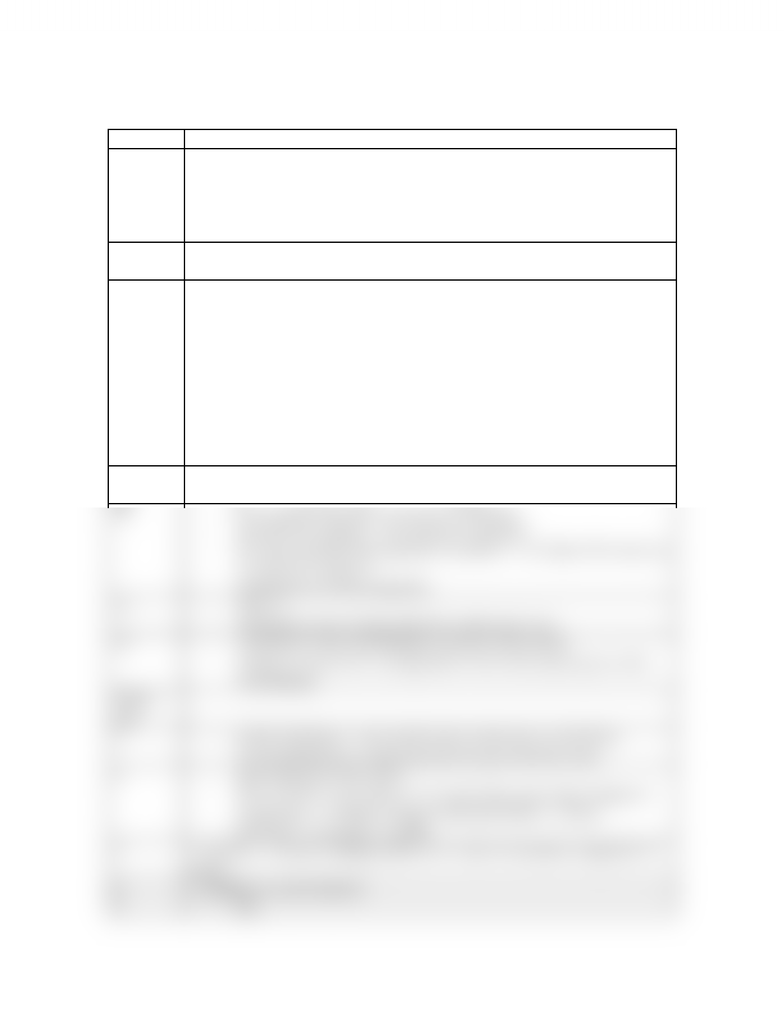# Notes taken during lecture

27 views2 pages
School
Department
Course
ProfessorLECTURE 12 – Thermodynamics
Slide No. Notes
3-q represents heat, or the transfer of thermal energy, which is another
type of kinetic energy
-restating delta E = -PdeltaV plus q, which q being equal to delta E
plus P delta V
-common kinds of reactions
7- the addition sign is circled because the negative sign for work has already
been included
8-Ex. A)
-A change in volume from 0.274 L to 448L
-W = -p times delta V = -(1atm)(448L-0.274L) = -447.7 Latm times
(101J/Latm)(kJ/1000J) = -45.2kJ
-Negative sign means energy lost, leaving system, so work is done on
surroundings
-Ex. B)
-W = -p times delta V = -1atm(5.6L)(101J/Latm) = -566J
-Ex. B) part 2
-Delta E = q + w = -(484kJ) + (-566J) = -484.6kJ
9 -What is absolutely true is B
-What could be true is all of them, so E
10 -heat capacity depends on amount of substance
-specific heat capacity – the amount is specified
-for water, specific heat capacity is around 2 – so it takes 2J to raise 1g
of water by 1 degree C
-metals have low heat capacities
11 -Ans: A
-Al transfers more energy, therefore melts more wax
12 -calorimeter is the surroundings; reaction is the system
-energy is conserved, so energy that is lost to the system goes to the
surroundings
NEXT
LEC
1-bomb calorimeter – how much water warms up or cools down
-in an insulated box so that any heat lost goes into the water
2-any energy goes into water
-EX. q of surr = q of water = C of water times mass times change in
temperature = 4.18J/gC (250g)(7.48C)(kJ/1000J) = 7.82 kJ
-Q of rxn = - q of surr = -7.82kJ
5- extensive – because enthalpy differs for 1 mole of reactants compared to 5
moles
6- enthalpy is a state function
8-EX.
www.notesolution.com
Unlock document

This preview shows half of the first page of the document.
Unlock all 2 pages and 3 million more documents.

## Document Summary

3 q represents heat, or the transfer of thermal energy, which is another type of kinetic energy restating delta e = -pdeltav plus q, which q being equal to delta e plus p delta v common kinds of reactions. The addition sign is circled because the negative sign for work has already been included. A change in volume from 0. 274 l to 448l. W = -p times delta v = -(1atm)(448l-0. 274l) = -447. 7 latm times (101j/latm)(kj/1000j) = -45. 2kj. Negative sign means energy lost, leaving system, so work is done on surroundings. W = -p times delta v = -1atm(5. 6l)(101j/latm) = -566j. Delta e = q + w = -(484kj) + (-566j) = -484. 6kj. Ex. q of surr = q of water = c of water times mass times change in temperature = 4. 18j/gc (250g)(7. 48c)(kj/1000j) = 7. 82 kj. Q of rxn = - q of surr = -7. 82kj. Extensive because enthalpy differs for 1 mole of reactants compared to 5 moles.

## Get access

\$8 USD/m\$10 USD/m
Billed \$96 USD annuallyHomework Help
Study Guides
Textbook Solutions
Class Notes
Textbook Notes
Booster Class